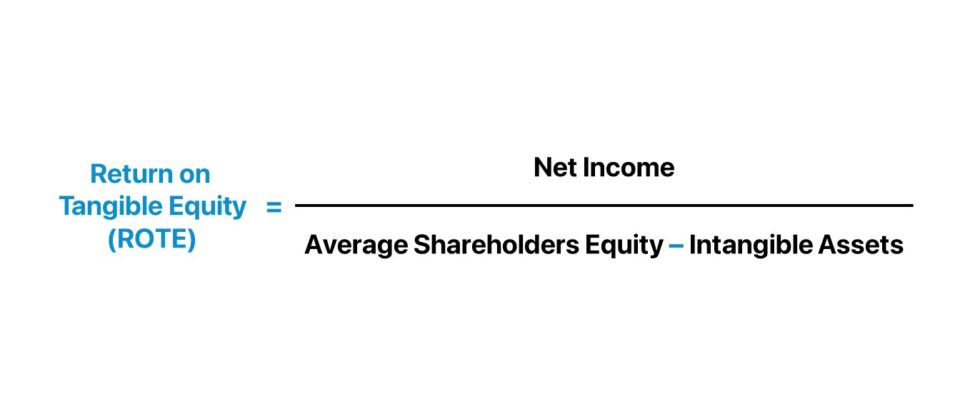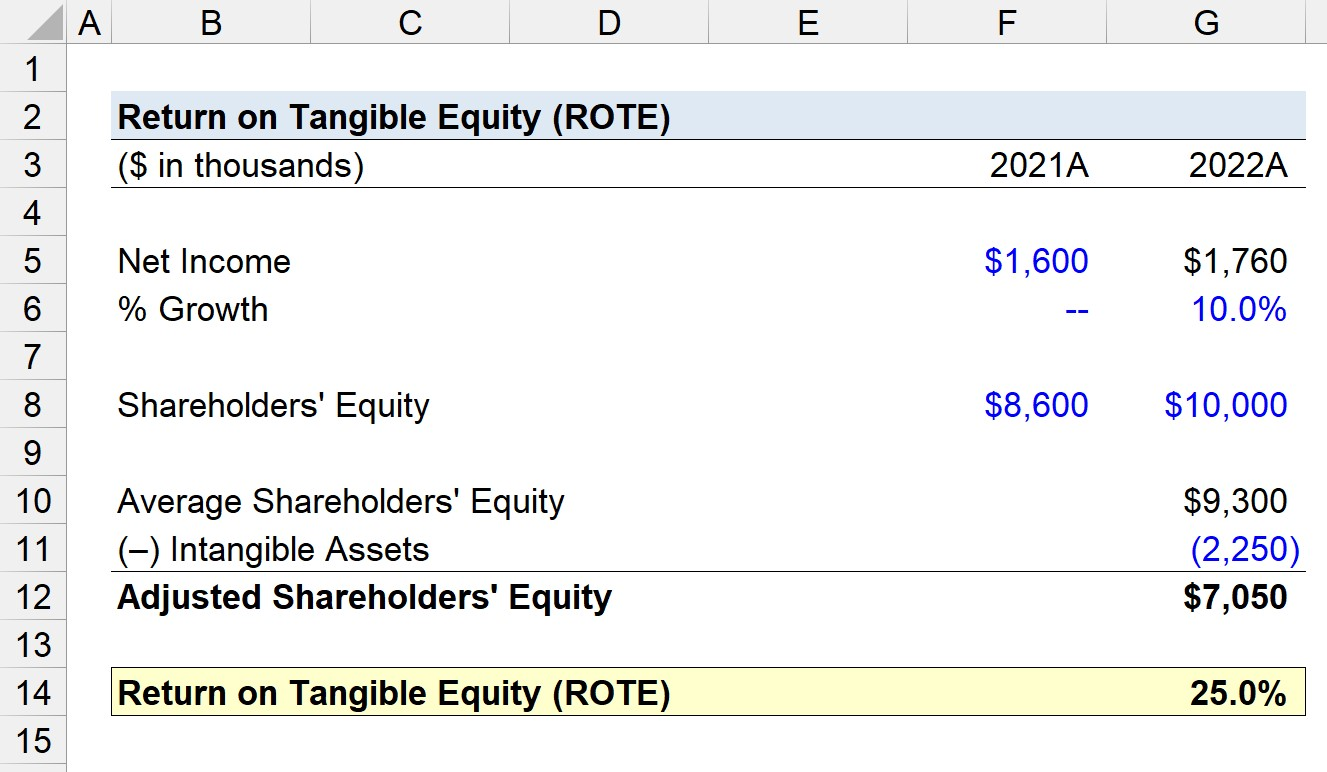Welcome to Wall Street Prep! Use code at checkout for 15% off.# Return on Tangible Equity (ROTE)

Guide to Understanding Return on Tangible Equity (ROTE)## How to Calculate Return on Tangible Equity?

The return on tangible equity (ROTE) is a method to determine the efficiency by which a company can generate profits using just its tangible assets.

The calculation of return on tangible equity (ROTE) consists of dividing a company’s net income (the “bottom line”) by its average shareholders’ equity, net of any intangible assets such as goodwill.

Conceptually, the return on tangible equity (ROTE) measures the implied return that shareholders anticipate receiving per dollar of tangible equity invested into the company.

Therefore, the more efficiently a company can spend its tangible assets to generate profits and create positive economic value on behalf of its shareholders, the more likely the company is to attain a sustainable, long-term moat.

The step-by-step process to calculate return on tangible equity is outlined as follows:

1. Determine Net Income → The net income metric is the post-tax earnings of a company after deducting all operating and non-operating costs and can be obtained from the income statement.
2. Calculate Average Shareholders’ Equity → The shareholders’ equity balance is recorded on the balance sheet, which unlike the income statement (and net income), is a “snapshot” in time as opposed to measuring performance across a time period. Therefore, the average balance of the shareholders’ equity account is used for the calculation to ensure alignment in timing.
3. Deduct Intangible Assets → Any intangible assets, such as goodwill, are subtracted from the average shareholders’ equity balance.
4. Calculate Return on Tangible Equity (ROTE) → The net income of the company can be divided by the adjusted average shareholders’ equity to determine the return on tangible equity.

## Return on Tangible Equity Formula

The formula to calculate the return on tangible equity is as follows.

Return on Tangible Equity (ROTE) = Net Income ÷ (Average Shareholders’ Equity Intangible Assets)

Where:

• Net Income → Net Income is the post-tax earnings of a company after deducting all expenses, including operating costs like cost of goods sold (COGS) and SG&A, as well as non-operating costs such as income taxes and interest payments. The net income metric represents the accounting profitability of a company, inclusive of all costs.
• Average Shareholders’ Equity → The average shareholders’ equity metric is the average balance of a company’s shareholders’ equity – as recognized on its books – across two periods. The shareholders’ equity line item includes the initial paid-in capital, retained earnings (i.e. the cumulative net income less distribution of dividends), and other capital contributions from shareholders. Thus, the shareholders’ equity metric is essentially a company’s net worth – i.e. total assets minus total liabilities – and measures the value of the collective equity stake.
• Intangible Assets → Intangible assets are non-physical assets that cannot be physically touched, contrary to assets like inventory. Common examples of intangible assets include patents, trademarks, copyrights, and goodwill. While a value is in fact ascribed to the intangible asset, the asset’s fair value cannot be easily quantified (i.e. more reflective of the control premium in M&A, which is influenced by various factors). To remove the value of intangible assets from the average shareholders’ equity, the stated value of the tangible assets is subtracted to focus solely on tangible assets.

The formula to compute the net income and average shareholders’ equity is as follows.

Net Income = Earnings Before Taxes (EBT) Taxes
Average Shareholders’ Equity = (Beginning + Ending Shareholders’ Equity) ÷ 2

The average shareholders’ equity balance of the company is determined by taking the sum of its beginning and ending balances and dividing by two, which is then adjusted for intangible assets.

Since the value of the intangible assets is readily available on the balance sheet of the company, there is no need for a manual calculation.

## Return on Tangible Equity (ROTE) vs. Return on Equity (ROE): What is the Difference?

• Return on Tangible Equity (ROTE) → By deliberately excluding the effects of intangible assets to isolate the company’s tangible equity, the return on tangible equity (ROTE) metric – at least in theory – offers a more accurate measure of a company’s capacity to generate returns from its tangible asset base.
• Return on Equity (ROE) → In contrast, the return on equity (ROE) – a fundamental measure widely recognized as a practical tool to measure the operating efficiency of a company – is virtually identical to the return on tangible equity, aside from the inclusion of intangible assets. Intangible assets, particularly those recognized for accounting purposes like goodwill, do not produce income, so the inclusion in the ROE metric could potentially distort the return. The difference between the two return metrics tends to widen for companies with significant amounts of intangibles sitting on their balance sheet.

## Return on Tangible Equity Calculator

We’ll now move to a modeling exercise, which you can access by filling out the form below.#### Excel TemplateSubmitting...

## Return on Tangible Equity Calculation Example

Suppose a company reported \$1.6 million and \$1.76 million in net income in fiscal year ending 2021 and 2022, respectively.

From 2021 to 2022, the company’s shareholders’ equity balance increased from \$8.6 million to \$10.0 million.

• Net Income, 2021A = \$1.6 million
• Net Income, 2022A = \$1.76 million (10.0% YoY Growth)
• Shareholders’ Equity, 2021A = \$8.6 million
• Shareholders’ Equity, 2022A = \$10.0 million

The average shareholders’ equity of our company comes out to \$12.65 million, which we must then adjust by our assumptions pertaining to the intangible assets balance.

Our company will be assumed to carry an intangible assets balance of \$2.25 million.

• Intangible Assets = \$2.25 million

After subtracting our company’s intangible assets from its average shareholders’ equity balance, we’re left with an adjusted shareholders’ equity balance of \$7.05 million.

• Average Shareholders’ Equity = (\$8.6 million + \$10.0 million) ÷ 2 = \$9.3 million
• Adjusted Shareholders’ Equity = \$9.3 million – \$2.25 million = \$7.05 million

Upon dividing our company’s current period net income by the adjusted shareholders’ equity balance, we arrive at a return on tangible equity (ROTE) of 25.0%, which implies that \$0.25 is generated per dollar of tangible equity invested.

• Return on Tangible Equity (ROTE) = \$1.76 million ÷ \$7.05 million = 0.25, or 25%Step-by-Step Online Course

### Everything You Need To Master Financial Modeling

Enroll in The Premium Package: Learn Financial Statement Modeling, DCF, M&A, LBO and Comps. The same training program used at top investment banks.